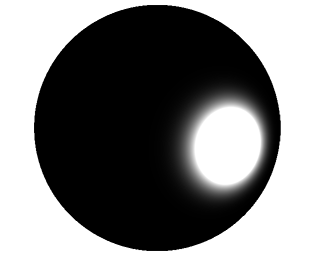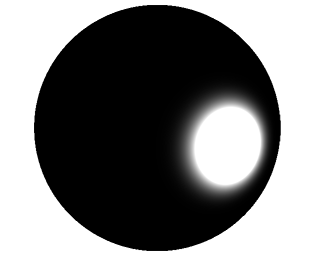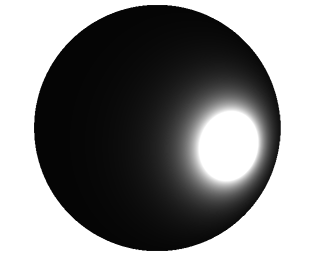# Chapter 71: Cook-Torrance

General form of the Cook-Torrance shading model:

$s$ in this equation is the specular material property, which we can use directly from our .pov files.

Diffuse reflection is often modelled using Lambertian:

There are other options, however, including Oren-Neyer.

Cook-Torrance specular reflectance (also sometimes simply called microfacet reflectance or microfacet BRDF) has the form:

$D$ is the Normal Distribution Function, which describes the concentration of microfacets which are oriented such that they could reflect light. (1)

$G$ is the Geometric Shadowing-Masking Function, which describes the percentage of microfacets which are neither shadowed or masked by neighboring microfacets. (1)

$F$ is the Fresnel Function, which describes the amount of light reflected by the material (as opposed to being absorbed or transmitted by the material).

## Symbols

$\vec n$ is the macrosurface normal.

$\vec l$ is the light vector.

$\vec v$ is the view vector.

$\vec m$ is the microsurface normal - in these computations (i.e. for implementation purposes) the half-vector is used.

$\theta_m$ is the angle between $\vec m$ and $\vec n$

$\alpha$ is the roughness constant. $\alpha$ is defined as such using the roughness material property from our .pov files: (4)

## Normal Distribution Functions

The $D$ function is always positive. (2) It is also normalized to satisfy certain geometric properties. (3)

This normalization is the source of the $\frac{1}{\pi \alpha^2}$ factors you see in the Blinn-Phong and Beckmann variations.

$D(m)$ is the concentration of microfacets oriented with subsurface normal $\vec m$. For our microfacet model we plug in $\vec h$ since we want $D(h)$, the concentration of microfacets which can reflect light from $\hat l$ to $\hat v$. (1)

### Blinn-PhongHere, $power$ is the “shininess” or “specular power”. There doesn’t seem to be a standard way to compute this from $\alpha$ or roughness. Past iterations of CSC 473 have used the reciprical of POV-Ray roughness:

I recommend (and use) the equation provided by Karis’: (4)

### BeckmannThis is the NDF which Cook and Torrance originally advocated for in (5).

Note that for normalized vectors, the following are equivalent:

And by trigonometric identity, the following are equivalent:

With those substitutions, you find the common form of the Beckmann NDF.

### GGX## Geometric Functions

### Cook-Torrance### GGX$\chi^+$ is the positive characteristic function:

## Fresnel Functions

### Schlick’s Approximation

2: Walter et al., Microfacet Models for Refraction through Rough Surfaces, 2007. Introduced the GGX form of D and G functions.

3: Nathan Reed, How Is The NDF Really Defined?, 2013. Describes where the normalization factor of D function comes from.

4: Brian Karis, Specular BRDF Reference, 2013. Reference of D, F, and G functions. Written by a UE4 graphics programmer.

5: Robert Cook & Kenneth Torrance, A Reflectance Model for Computer Graphics, 1982. Original Cook-Torrance paper. Many of the equations in this paper have slightly different forms in modern usage, so beware.